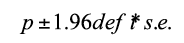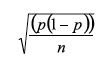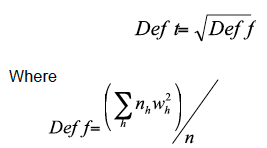# Patient Experience survey of GP and local nhs services 2011/12 Volume 1: Technical Report

Scottish Patient Experience Survey of GP and Local NHS Services 2011/12. This is a postal survey which was sent to a random sample of patients who were registered with a GP in Scotland in October 2011. This report contains details the survey design and development.

### Annex D: Calculation of confidence intervals

The 95% Confidence Intervals for NHS Boards in Table 13 were calculated using the following formula:The s.e (standard error) was calculated as:Where:

p= point estimate (i.e. proportion who answered positively)

n= achieved sample size (i.e. number who answered the question).

The design effect factor was calculated as:Wh= weight given to each stratum h, where each GP practice was a stratum

n = achieved sample size (i.e. number who answered the question).

Example of calculation of confidence interval for NHS Ayrshire and Arran (Table 13)

For NHS Ayrshire and Arran, where the result (proportion of patients rating the overall rating of care provided by GP Surgery as excellent or good) is equal to 88%, and the total sample size is 7,878.

Then p = 0.88, n = 7,878

SE = √(p(1-p))/n

= √((0.88(1-0.88))/7,878

= 0.003708

deft= 1.118110

95% Confidence Interval (CI) = 1.96 x deft x SE

CI = 1.96 x 1.118110 x 0.003708

= 0.008126

Therefore the CI, expressed as a percentage (i.e. multiplied by 100) is ±0.81%

The 95% confidence interval for NHS Ayrshire and Arran, where the proportion is 88%, is 86.8% - 88.5%.

### Contact

Email: Gregor Boyd